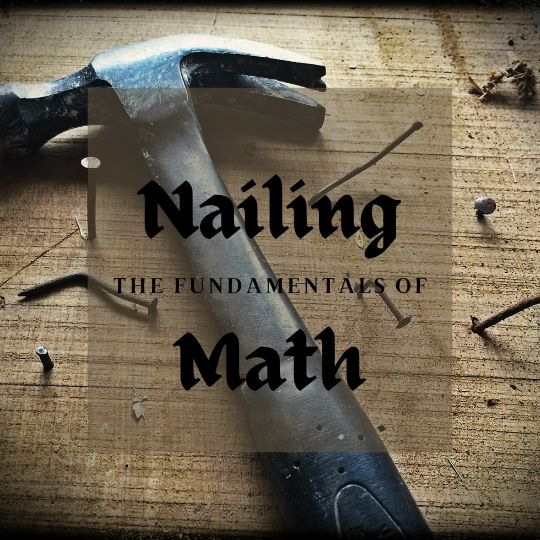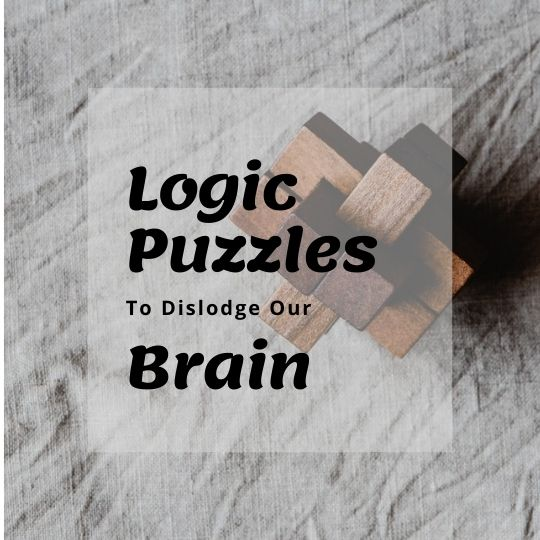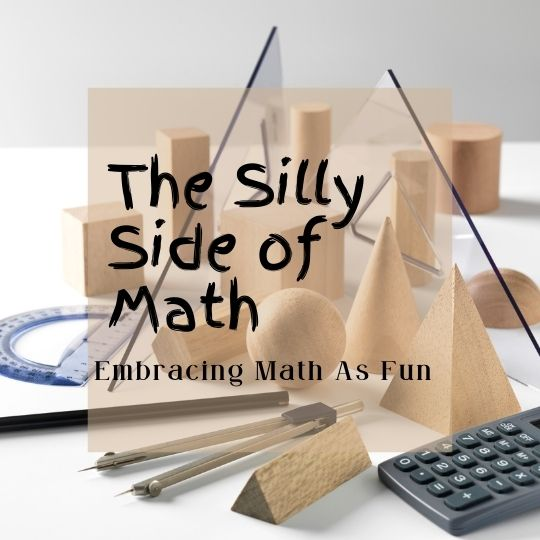# Math, Logic & Strategy### Basic Elementary Math

Numbering, Addition, Subtraction, Muliplication, & Division

Browse Math Printables

### Basic Elementary Math

Numbering, Addition, Subtraction, Muliplication, & Division

Browse Math Printables

### Basic Elementary Math

Numbering, Addition, Subtraction, Muliplication, & Division

Browse Math Printables

### Basic Elementary Math

Numbering, Addition, Subtraction, Muliplication, & Division

Browse Math Printables

# In Math, Everything Builds One Another, So It’s Important To Get The Basics Down.

##### Number Properties and Operations

Sequence

Estimation

Ratios / Proportional reasoning

Fractions

##### Basic Mathmatical Operations

Multiplication / Division

##### Measurement

Capacity

Weight/mass

Time

Temperature

Geometric Operations (length, area, and volume)

##### Algebra

Equations / Inequalities

pattern / relations

functions

algebraic representation

variables / expressions / operations

simple patterns and expressions

##### Geometry

Video Reference

Worksheets

Solving Equations

##### Data Analysis and Probability

data representation

characteristics of data sets

experiments and samples

probability

median / range / mode

Statistics# Beyond The Classroom: The BEST Math Tools

### Junior Front End Developer

Extra Space – San Jose, California – View Listing

### Product Manager

Elegant Themes – San Francisco, California – View Listing

### UX Researcher

Divi Corner – San Francisco, California – View Listing

# Featured Articles## How to Find a Fantastic Tutor## Embrace the Fun Side of Math